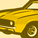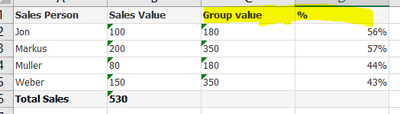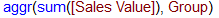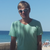Announcements
You can succeed best and quickest by helping others to succeed. Join the conversation.
cancel
Showing results for
Did you mean:Creator

## AGGR in Chart

Hi, I have a table like thisI want to have report like thisbut i am not able to get the proper result in QLikView report.

Qlikview reporti am using following expressions for Group value =if i write the expressions as Calculated dimension. that works fine. but with that i can not get  %. I can do group by in Load script but i want to do it in Chart. Is it possible? Any suggestion will be highly appreciated.

Thank

Kazi

Labels (3)

• ### Scripting

1 Solution

Accepted SolutionsMaster II

Dimension:

``Sales Person``

Exp for "Group value as Expression"

``sum(aggr(sum(Total <Group> [Sales Value]), Group,[Sales Person]))``

Exp for "%":

``sum([Sales Value]) / sum(aggr(sum(Total <Group> [Sales Value]), Group,[Sales Person]))  ``Master II

Dimension:

``Sales Person``

Exp for "Group value as Expression"

``sum(aggr(sum(Total <Group> [Sales Value]), Group,[Sales Person]))``

Exp for "%":

``sum([Sales Value]) / sum(aggr(sum(Total <Group> [Sales Value]), Group,[Sales Person]))  ``Community Browser